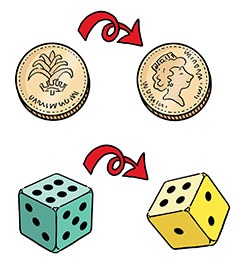Assessing risk in engineering, work and life

Start this free course now. Just create an account and sign in. Enrol and complete the course for a free statement of participation or digital badge if available.

Free course

# 3.2 Calculating probability

Coins and dice provide good examples for considering probability, as there is a limited but well-defined number of possible outcomes in each case (Figure 14).Figure 14 Tossing coins and rolling dice

## Finding the probability of a particular outcome

To calculate the probability of a particular outcome from an event:

1. Count the total number of possible outcomes.
2. Count the number of times the outcome of interest occurs.
3. Express the two numbers as a proportion, a fraction, a decimal or a percentage in its simplest form.

For example, if a person tosses a coin, there are two possible outcomes: ‘heads’ and ‘tails’. If the coin is ‘fair’ and has not been tampered with, these outcomes are equally likely. So the probability that a single toss gives ‘heads’ can be described as 1 in 2, , 0.5 or 50%.

In standard mathematical notation, the probability of a particular event happening is expressed as

where means that the value of lies in the range 0 to 1. So the probability of tossing a coin that lands showing ‘tails’ would be written as .

T193_1